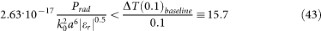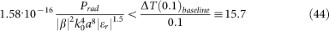## Introduction

Ever since the first endoradiosonde1 was demonstrated in 1959, remotely monitored implanted medical devices have been one of the key paradigms in biomedical science and engineering. A small enough, unobtrusive, responsive sensor capable of witnessing biological processes at the smallest of scales would support a wide range of biomedical research and therapy applications. Advances in neuroscience and the success of semiconductor electronics in following Moore's Law to below the 30 nm node suggest the possibility that the remotely addressable device may finally be able to reach the scale at which individual neurons can be interrogated and reported upon. Indeed, the advent in 2007 of the nanoradio, with its micron-sized antenna2, has led to speculation as recently as 2012 that in vivo RF wireless nano networks3 may soon be possible.

We show in this contribution that a conventional RF telemetry link depending on antennas smaller than 60 microns transmitting from within the human brain is rendered unfeasible by fundamental physical limitations.

## Results

### Why RF?

Implicit in the implanted sensor application is the requirement for the telemetry stream to negotiate the propagation properties of living tissue. A variety of modalities have been explored for transmitting energy and information through biological media, including: magnetic fields (MRI4 and near-field powered devices5), microwave6, ultrasound7,8, Infrared9 and photonic10. The exacting requirements of magnetic field strength and uniformity for micro-MRI are well documented and in general limit the method to the examination of small biological volumes that can be contained within the controlled field environment. The rest of the modalities are based on wave propagation and in principle could interrogate large volumes remotely. However they all must deal with the multiple scattering and loss effects of realistic biological media that significantly limit the depth of signal penetration.

The propagation loss through lossy media is modeled as exp(−2αd) where d is the distance the signal propagates and ‘α’ is the attenuation coefficient for the propagating field calculated from the complex permittivity of the media. When the scale of the inhomogeneities in the medium is larger than the wavelength, the effective attenuation constant may be considerably larger than this intrinsic attenuation constant due to multiple scattering effects. This is the case with optical scattering within tissue and optical propagation in turbid media11. However, when the wavelength is much larger than the local inhomogeneities, the dominant attenuation mechanism remains the medium's intrinsic absorption coefficient. To first order this is the case with radio frequency microwave modalities operating below 3 GHz (free space wavelength λ0 > 10 cm.) Capable of penetrating through many centimeters of body dielectric, these have always been considered prime candidates for embedded telemetry applications.

The above considerations motivated much of the initial speculation over the nanoradio2, a mechanical oscillator consisting of a vibrating carbon nanotube carrying electric charge at the tip of the tube and therefore capable of translating its mechanical vibrations into a radiated electromagnetic signal. Unfortunately, analyzing the published data on the system to compare the mechanical power expended to the electromagnetic power radiated (e.g. following Ref.12) it is easy to show that a typical cantilever dissipating 15 · 10−12W of mechanical power only radiates about 4.5 · 10−27W of electromagnetic power. That is, the efficiency of this transmitter is −155 dB without yet accounting for the loss of electromagnetic power that would be suffered in the body dielectric. This is of the same order of magnitude as the attenuation faced when attempting to communicate from land to a submarine at the bottom of the sea over a distance of 100 km. In 1990, Chave13 concluded that communication under such conditions could be accomplished but only at a frequency of 1 Hz (ULF) at a rate of 3.5 bits/sec. The nanoradio is not a realistic implantable medical device. Nevertheless its existence presses the question, could an RF transmitter be made small enough to allow neuron by neuron telemetry from the human brain?

For conciseness, the various examples and equations in the body of this report address the specific frequency that is the best choice for each case considered (human and rodent). This optimum frequency value is the frequency at which minimum heat is dissipated in the biological tissue by an embedded dipole. It has been calculated by combining the effects of the near field power dissipation of the antenna, the antenna efficiency and interactions with the tissue permittivity, all calculated as a function of frequency. (The derivations in the Methods section are included to enable the reader to replicate these results.) In the case of the human subject this value is 2 GHz and for the rodent it is 3 GHz.

### The size of the Problem for the human head: 6 orders of magnitude

Although neurons range in size from 4 to 100 microns, the recording of electrical signals from an identifiable individual neuron will require sampling of regions of the order of the axon hillock, that is, smaller than 1 micron across. For this reason we would expect the maximum size of a device capable of detecting and reporting on an individual neuron to be no more than 10 microns across (the net diameter of the nanowire based sensor in Ref.14).

The root cause of the problem faced by micron sized antennas is the fact that electrically small antennas (size < λ/8) store orders of magnitude more energy per cycle in their near field than the power they can radiate to the far field. This ratio is known as the Quality factor of the antenna. According to the Fano-Chu limit15,16,17,18,19 for an antenna that fits within a sphere of radius, a, the Q is at least of the order of 1/(ka)3, where k is the propagation constant in the medium, 2π/λ. For a 20 micron antenna in the body dielectric at 2 GHz this ratio is of the order of 51 million; for an 80 micron antenna it is of the order of 800,000. Since all realistic materials are lossy, the enormous amount of energy available per cycle in the near field ends up being consumed as heat in the body and materials of construction.

For a first order calculation, assume the human head can be represented by a sphere 5 cm in radius within which the embedded spherical RF antenna (of radius a) must function. The real-time reporting of neuronal activity is estimated to require communication rates of the order of 300 Kbps. Given a mean neuron spike duration of 1 ms and the desire to track a spike train of at least 15 spikes, the typical time window of data is 100 ms long20,21,22. We will assume this is the length of the typical telemetry stream we wish to transmit at any given time. Using 300 Kbps as the required Channel Capacity, Cmin and assuming a carrier bandwidth of at most 2*Cmin, Shannon's equation23 tells us the theoretical minimum power required to communicate in the presence of Thermal noise at room temperature (300 K):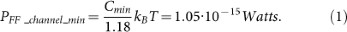However, to actually acquire the information with a realistic receiver three additional factors must be taken into account: (i) The information must be encoded in such a way that errors can be controlled. Assuming a maximum Bit Error Rate (BER) of 10−3 is acceptable, one of the simplest coding schemes, On-Off-Keying (OOK), requires a Signal-to-Noise Ratio (SNR) of +7 dB. The more robust Binary Frequency Shift Keying (BFSK) for the same BER of 10−3 requires a SNR of 10.5 dB7,24. (ii) To detect the faint signal at the receiver, amplifying stages are required. The noise contribution of these and all other impairments in the receiver result in what is called the system's Noise Figure (NF). Individual amplifiers with NF of +2 dB are possible24 yet the typical NF for wireless receivers ranges from 5 dB to 14 dB. (iii) A Link margin is needed to account for contingencies. In a well-controlled engineering system even under the best of circumstances we would assume at least a +3 dB margin. In a system where failure can lead to catastrophic results, as in life support devices, +20 dB is probably mandatory in addition to the elimination of any single-point failures.

Given the desire to receive signals from cohorts of wireless neuronal transmitters potentially interfering with each other +10 dB is an appropriate assumption for SNR, +3 dB is a defensible total receiver NF25 and +6 dB is probably the lowest acceptable link margin. The result is that the realistic minimum power to carry out the telemetry at 300 Kbps is: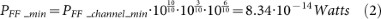The question then is: What is the power output required from the embedded antenna? The farthest the antenna can be from the surface is 5 cm, the closest, the thickness of the skull. So, assuming a typical distance of 3.5 cm from the antenna to the nearest point on the surface and given the properties of the body dielectric at 2 GHz (the frequency of minimum power dissipated) the spreading of the wave (space loss) and the attenuation by the complex dielectric constant of the body combine to contribute −38.9 dB loss to the signal received by an external antenna at the surface. Therefore the embedded antenna must make up these losses and radiate towards the surface a total power of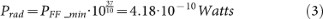of the order of half a nano Watt.

This may seem to be a minute amount of power, except that, as mentioned, for electrically small antennas immersed in a lossy medium the radiated portion of the power is a very small fraction of the total power dissipated into the medium and the materials of construction. In most engineering applications this is not a problem because all we would have to do is increase the power input into the antenna until we attain the desired power radiated. But in the case of a telemetry system reporting living cell activity, this is not necessarily possible. Neither the power dissipated into the body nor the temperature rise of the antenna can be allowed to alter the system we are trying to observe. This means the maximum power that can be input into the antenna is limited by safety margins.

Based on FCC guidelines for microwave SAR deposition and recent mouse experiments26,27 we assume the safety limit for power deposited in the body must be less than 0.1 W/Kg. When dealing with electrically small antennas, we have only two choices, either an electric dipole or a magnetic dipole (traditionally a loop antenna). Figure 1 illustrates a plausible configuration for the antennas, both fitting within a sphere of radius a and assuming half the volume is devoted to the transmitter electronics. Thus, for the sake of simplicity, we consider the ideal practical scenario where the sensor is completely embedded within the antenna, understanding that its size does not constrain the size the antenna.

The power dissipated into the body tissue around the antenna is calculated as the integral of the antenna's near field ohmic loss (Joule heat dissipated into the electrical conductivity of the body.) In this respect the magnetic dipole has an advantage because the electric field it produces in the environment is farther spread out than the electric field of the dipole, thus reducing the induced current density. While the electric dipole dissipates 90% of its heat within a sphere of radius 2.2a, the magnetic dipole does so within a sphere of radius 10a. Using the volumes of these spheres as the averaging volumes for the SAR calculation and assuming a brain mass density of 1000 Kg/m3 we can cast the SAR safety limit in terms of the power radiated (refer to Methods, equations (30) and (31)):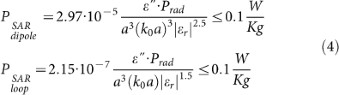where εr is the complex dielectric constant of the body, ε" its imaginary (loss) part and k0 is the propagation constant in free space. We see from the appearance of a power of the radius of the antenna in the denominators that this safety limit will constrain how small we can make the antenna.

The second safety limit is derived from the accepted thermal damage time-and-temperature threshold model, CEM4328. The CEM43 model sets the damage threshold for a duration of exposure of δt at CEM43 = δt · R(43−T) ≥ 1 min, where the base R is 0.25 for T < 43°C and 0.5 for T > 43°C. Setting the brain temperature at 36.5°C and choosing a safety factor of 10 from the damage threshold, the limit temperature rise, ΔT, as a function of time in seconds is: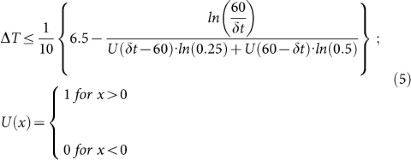Assuming that the temperature rise in the antenna will be much faster than in the surrounding body (given the limited thermal conductivity and the specific heat of the body as compared to metals) we can estimate the rise in temperature of the antenna (and therefore at the interface between the antenna and the body) once we have the Power dissipated as heat in the metal of the antenna: Pmetal = 0.5 · I2Rmetal. Although both a realistic electric dipole and a realistic magnetic dipole may have approximately the same metal resistance, Rmetal, the electric dipole has the advantage in this case because an electric dipole is essentially an open circuit while the loop antenna is a short circuit. When radiating equal amounts of power, the current flow in the loop antenna far exceeds that in the electric dipole. Again we cast the power dissipated in terms of the power radiated (refer to Methods, equations (41) and (42)).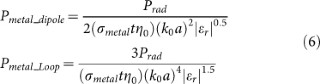where the electric conductivity of copper is σmetal = 5.8 · 107S/m and the thickness, t, of the antenna conductor is assumed to be a/5. The lower power of a in the denominator in case of the dipole emphasizes its advantage.

The rise in temperature follows from the definition of the heat capacity and the fact that the heat generated internally is Pmetal*δt: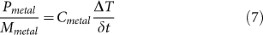where Mmetal is the mass of the (copper) antenna =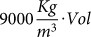and Cmetal is the specific heat capacity (of copper) =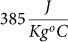. Calculating the change in temperature ΔT over the time δt = 100 ms, we obtain our second safety limit (refer to Methods, equations (43) and (44)):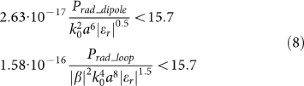Given these results we can solve the safety limit equations for the radius, a, of the antenna that meets the communication channel requirements yet does not alter or damage the very environment we are trying to measure.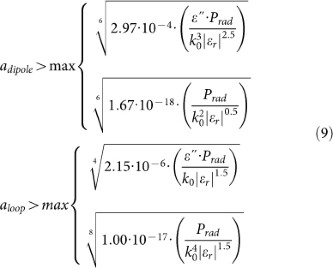Table 1 summarizes the results at 2 GHz (the best case) for the human head scenario with the receiver antenna assumed to be at the surface of the head. The table also includes the case of a loop with a permeable core that can increase the loop's dipole moment by up to a factor of 3 and the total power consumed, Prad + PSAR + Pmetal. None of these antennas is close to the target maximum size of 10 microns.

If the receiver were placed 1 meter away we would have to account for space loss through the air. However, we could also use a parabolic dish or horn receive antenna to increase the gain at the receiver. Assuming such an antenna ½ a meter on the side the best carrier frequency is now about 1.2 GHz. The results for this case are summarized in Table 2.

The natural question to ask is, what kind of telemetry bandwidth would be supported by a 10 micron diameter antenna? Following the same procedure without fixing Cmin at 300 Kbps leads to equations 10 and the plots in Figure 2.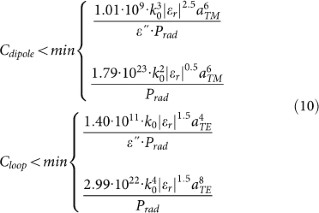Even in the best case (dashed black curve in the figure, the loop with a magnetic core) an 11 micron diameter antenna communicating to a receive antenna at the surface of the head could only achieve a channel capacity of 0.3 bps. This is 6 orders of magnitude below our desired rate. (In this case the telemetry stream would have to be almost 3 seconds long to allow 1 bit per stream.)

### The rodent model case

In the case of a rodent experiment we model the brain as a sphere 1 cm in radius and assume the receiving antenna is 10 cm away. Since the wavelength in the body is of the order of 2 cm the surface of the mouse head is in the near field of the antennas so no space loss is assumed through the mouse head. There is still attenuation due to the complex dielectric constant of the brain (about −4 dB) and there is space loss to the receiver through the 10 cm of air of the order of −18 dB. However we can then assume a receive antenna approximately 1 foot square whose gain would recover 12 dB. Thus the net loss of power from the embedded antenna to the receiver is about −10 dB (a factor of 10). Therefore for the rodent experiment the required power radiated at the antenna is equation 11.We have kept the assumptions of receiver NF, SNR and Link Margin the same as for the human case. For the rodent experiment the minimum power dissipation occurs at around 3 GHz. The results are summarized in Table 3.

We are much closer to the size estimated to be required for single neuron reporting. However, the penalty in bandwidth (channel capacity) for miniaturizing further is still severe. In this case a 14.5 micron loop antenna with a magnetic core (dashed black curve in Figure 3) or 19 microns conventional loop antenna (blue curve) could support a 3 Kbps telemetry channel. Though this is 2 orders of magnitude below the desired 300 Kbps and though the antenna would be 50% to 100% larger than the estimated maximum size of 10 microns this is probably the only workable scenario for testing the concept of in vivo neuronal RF telemetry.

## Discussion

The acknowledged weak point of the present model is its simplified view of the temperature rise of the antenna. This is especially crucial because the magnetic dipole loop antennas pose a minimal SAR risk and yet because they draw so much current they quickly run up against the thermal damage threshold. The subject of heating of local tissue by implanted devices is gaining renewed attention as these devices get smaller and more powerful29,30. A time dependent heat equation solution of the problem of the radiating antenna including heating of its materials, heat also generated by the transmitter electronics and the heat transfer properties of any proposed thermal insulation (to attempt to protect the body) is the next necessary step to determine the firmness of the limiting boundaries established here. Perfectly insulating the antennas from the body, even if it were possible, is not necessarily the ideal solution since the thickness of the insulation will increase the size of the antenna/sensor and will have the unintended consequence of raising the temperature of the transmitter's onboard electronics. A ΔT of 40°C in the internal electronics could exceed most miniaturized devices' operating temperature.

## Methods

To establish the fundamental limitations encountered by embedded antennas we begin with the most general spherical antenna model. Since the antennas of interest are electrically small (radius a λ in the medium) we find this problem has already been addressed in the classic literature in the context of antenna efficiency15,16,17,18,19. The result is that in determining the limiting behavior there is no reason to consider any modes higher in order than the two fundamental dipole modes.

Every spherical mode supports in its immediate environment a reactive near field consisting of the non-radiated electromagnetic energy oscillating per cycle in the external medium around the antenna. For aλ, the higher the order of a mode, the higher is the ratio of this near field energy per cycle to the radiated power. As a result, the higher the order of the mode the higher the proportion of power deposited into the body and the antenna's structure. Since we wish to minimize this power deposition into the body and antenna and since the spherical modes are orthogonal, there is no way of limiting this energy except by selecting the two lowest modes. These are called the electric dipole (TM) and magnetic dipole (TE) modes.

Now we add a bit more engineering reality. The assumption of a spherical antenna occupying the entire volume available within a is fallacious since the transmitter circuitry itself must occupy some volume. Furthermore the transmitter structure will affect the antenna's performance because the antenna's near field will induce currents on the transmitter package and these currents will interfere either constructively or destructively with the antenna's primary currents. Therefore we propose that an appropriate model for real antennas would take either of the forms suggested in Figure 1 where one half of the volume is assumed to be the “sealed” payload and contains the rest of the transmitter equipment plus the assumed neuronal sensors and so forth. In the case of the electric dipole (TM antenna) the lower hemisphere is the payload; in the case of the loop antenna (TE antenna) the left half is the payload. As suggested in the figure, the antenna current is excited by a voltage source that raises the antenna element to a voltage, V, relative to the payload which then serves as the counterpoise or ground. (If we acknowledge from the outset that the antenna will be much larger than the sensor package and transceiver then the equations for a full dipole and a full loop can be easily substituted and used instead of the “half” versions used here.)

### Determining the minimum power to be radiated by the antenna

Under the lowest order modes assumption, we know the complete electromagnetic field outside the antenna, at a distance r > a, is either:

TM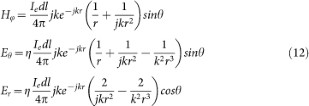Or TEwhere Iedl and Imdl are the electric and magnetic current moments of the antenna, ω is the radian frequency, k is the propagation constant in the medium given by, with k0 being the propagation constant in free space k0 = 2π/λ and εr the complex relative permittivity of the body. The wave impedance of the medium is, with η0 being the impedance of free space 377Ω. The radiated power density in the far field is then:Therefore the total power radiated to the surface of the head at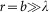is obtained by using only the 1/r terms of the transverse electromagnetic fields in the above expressions: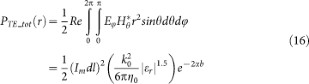where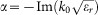, is the attenuation constant of the body dielectric. Of this total power radiated only a fraction is captured by an external antenna.

Assuming the external receive antenna is a dipole located close to the head (but not close enough that it incurs loss from the head's dielectric properties) the captured power can be determined by converting the total power radiated to power density by dividing by 4πb2, the surface area of the head sphere and then multiplying by the capture cross section of a dipole which is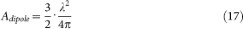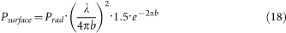Here (λ/4πb)2 is called the space loss, 1.5 is the Directive Gain of the receiving dipole and e−2αb is the loss due to attenuation in the medium. The Psurface required is determined from Shannon's channel capacity in the presence of room temperature thermal noise. However, as discussed before, engineering reality requires additional power to limit the Bit Error Rate through some coding scheme, compensate for the Noise Figure of the receiver electronics and supply a Link Margin. These were assumed to be 10 dB, 3 dB and 6 dB respectively. Therefore the true power required to be radiated at the transmit antenna is: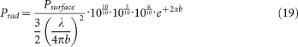From these it follows that we know the minimum current moments of our two possible modes: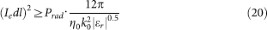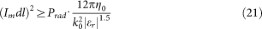### The Power dissipated in the body

The power dissipated in the body to be compared to the SAR limit is the power due to the induced electric currents in the conductivity of the body, where σ = ωε0ε" and ε" = −Imr). By the definition of SAR this ought to be an average power. Since SAR guidelines are based on assuming an external source, the minimum volume for averaging this power is given as 1 cubic centimeter. However we have an internal source which in principle could be occupying a volume as small as 1 cubic micron. To translate the SAR limit fairly we average the power deposited over the volume in which 90% of that power is deposited. That is, we must perform an integral of the form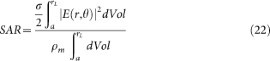where rL is the radius at which 90% of the power has been deposited and ρm is the mass density of the body dielectric; a good approximation is 1000 Kg/m3.

As a best case (most forgiving) scenario we calculate this deposited power using the near field of the antenna (the 1/r2 and 1/r3 terms in the electromagnetic field expressions) since the power lost by the 1/r radiated wave can always be recovered from the e−2αb term of the far field expression. Because the skin depth (1/α) in the body is larger than 10 mm at all frequencies of interest (below 4 GHz) the exponential term's contribution to the near field power can be neglected. Thus as a best case we ignore it and render trivial the determination of rL. It can be shown that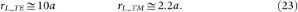Therefore,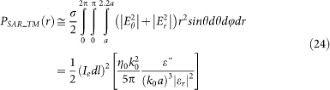to be averaged over the volume,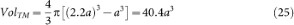For TE: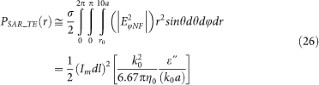to be averaged over the volume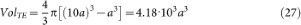Immediately we can substitute equations (20) and (21) and express the power dissipated into the body in terms of the minimum far field power: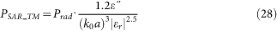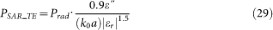Since the quantity k0a is very small, equations (28) and (29) show the well-known result that the small elementary TE mode radiator (magnetic dipole) dissipates less power into a surrounding conducting medium than the small TM mode radiator (electric dipole) of the same size. Using the averaging volumes we conclude that: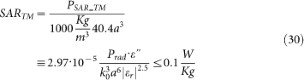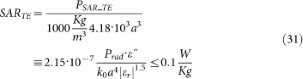with a in meters and k0 in rad/m. Equations (30) and (31) constitute our first safety constraint.

A good approximation below 3 GHz to the FCC accepted model for the human head is the relative permittivity given by the following multi-Debye relaxation model including a DC conductivity of 0.68 S/m (with the frequency written in GHz):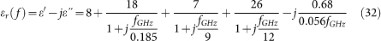where ε′ and ε" are respectively the real and imaginary parts of the dielectric constant of the material.

### The temperature rise of the antenna

The dimensions chosen in Figure 1 ensure the two antennas fit within the same volume. If as a best case we treat the counterpoise for the loop as a full ground plane, the method of images ensures that the effective magnetic current moment of the loop shown is,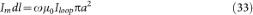In the presence of a permeable core of specific polarizability β this is increased to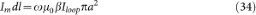For a spherical permeable core inside a non-magnetic medium, the specific polarizability is given by31: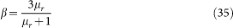For large real permeabilities its maximum value is 3.

The current moment of the electric spherical dipole is: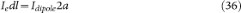and the respective resistances are approximately: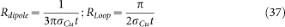with σCu the conductivity of Copper and t the thickness of the hemispherical shell for the dipole and thickness of the conductor in the case of the loop. Since the power dissipated in this resistance is given by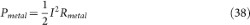It follows that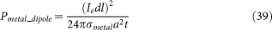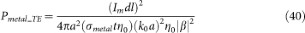where t is assumed thinner than the metal skin depth at all frequencies. Again using equations (20) and (21):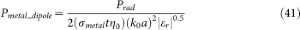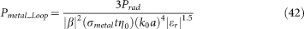The primary job of the permeable core can be seen in equation (42); it drops the power dissipated into the metal of the loop. Because the core increases the magnetic dipole moment of the loop, it requires less current to attain the same power radiated. (For now we ignore the loss in the permeable core by assuming that a material with μr > 20 and μ" < 2 is available at the required frequency.)

The volume of both antennas is approximately 2πa2t, so that their mass is approximately 9000*2πa2t. Dividing the power dissipated in the metal by this mass and the heat capacity of copper and by setting t = a/5 we obtain the second of the safety limits: